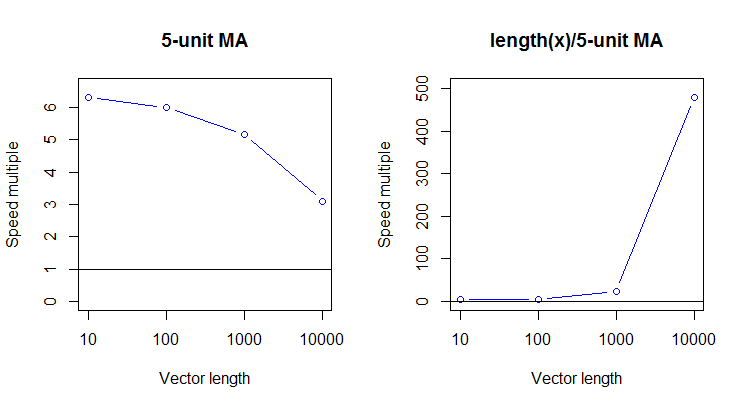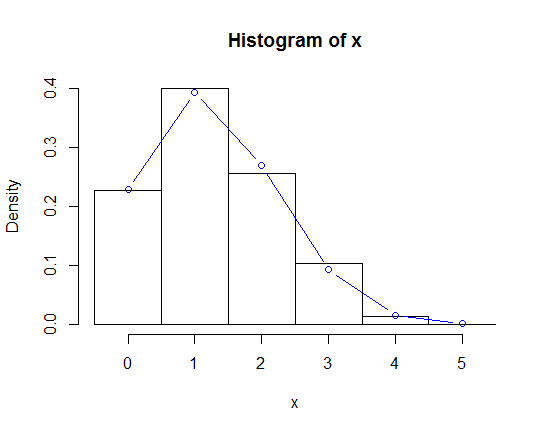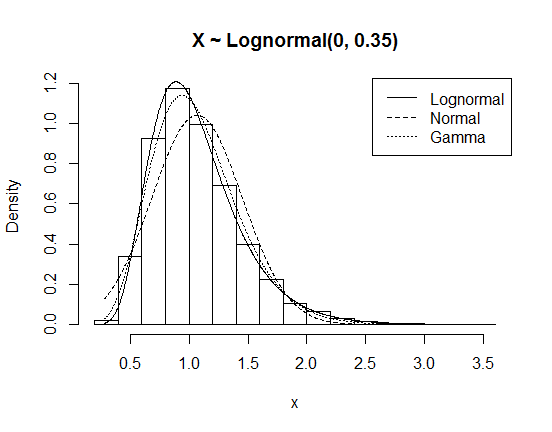# Convenience Functions, Moving Window Statistics, and Graphics

## Introduction

This package contains:

1. Functions that do something convenient.
2. Functions for calculating moving-window statistics.
3. Functions for generating graphs.

## Convenience functions

### truerange

The base R function range returns the minimum and maximum of a vector, but the “range” is actually defined as the difference between the minimum and maximum. This function calculates the actual range. It is equivalent to the base R code diff(range(x)), but a bit simpler and much faster.

x <- rnorm(1000)
all.equal(diff(range(x)), truerange(x))
#>  TRUE
as.data.frame(print(microbenchmark(diff(range(x)), truerange(x), times = 500)))
#> Unit: microseconds
#>            expr    min     lq      mean median     uq     max neval
#>  diff(range(x)) 17.369 18.948 30.542008 30.987 35.132 172.894   500
#>    truerange(x)  2.764  4.342  6.611704  5.527  7.106 166.973   500
expr min lq mean median uq max neval
diff(range(x)) 17.369 18.948 30.542008 30.987 35.132 172.894 500
truerange(x) 2.764 4.342 6.611704 5.527 7.106 166.973 500

### bmi3, bmi4

It isn’t hard to create body mass index (BMI) groups from continuous BMI values, but it is hard to remember how BMI values on the cutpoints get classified. The cutpoints according to the CDC are:

BMI values Classification
< 18.5 Underweight
[18.5, 25) Normal weight
[25, 30) Overweight
>= 30 Obese

The function bmi3 creates 3 groups (lumping the first two above into “Normal weight”), while bmi4 creates 4 groups. Both return factor variables, with or without labels depending on labels.

bmi <- round(runif(100, min = 15, max = 45), 1)
table(bmi3(bmi))
Normal weight Overweight Obese
27 22 51
table(bmi4(bmi, labels = FALSE))
[-Inf,18.5) [18.5,25) [25,30) [30, Inf)
13 14 22 51

### sumsim

This function creates tables summarizing results of statistical simulations, providing common metrics of performance like mean bias, standard deviation, mean standard error, mean squared error, and confidence interval coverage.

To illustrate, suppose $$X_1, ..., X_n \sim N(\mu, \sigma^2)$$, and we wish to compare two estimators for $$\sigma^2$$: the MLE ($$n$$ in denominator) vs. the sample variance ($$n-1$$ in denominator).

MLE <- c()
s2 <- c()
for (ii in 1: 1000) {
x <- rnorm(n = 25)
MLE[ii] <- sum((x - mean(x))^2) / 25
s2[ii] <- sum((x - mean(x))^2) / 24
}
kable(sumsim(estimates = cbind(MLE, s2), truth = 1))
Mean bias SD MSE
MLE -0.036 0.277 0.078
s2 0.004 0.289 0.083

You can request different performance metrics through the statistics input; some of them, like confidence interval coverage, require specifying ses with standard errors.

## Moving window statistics

### moving_mean

The function moving_mean is one of dozens of moving average functions available in R. I’m not sure it’s the absolute fastest, but it is much faster than roll_mean in RcppRoll.

library("RcppRoll")

lengths <- c(10, 100, 1000, 10000)
multiples1 <- multiples2 <- c()
for (ii in 1: 4) {
n <- lengths[ii]
x <- rnorm(n)
medians <- summary(microbenchmark(roll_mean(x, 5), moving_mean(x, 5),
roll_mean(x, n / 5), moving_mean(x, n / 5),
times = 50))\$median
multiples1[ii] <- medians / medians
multiples2[ii] <- medians / medians
}
par(mfrow = c(1, 2))
plot(1: 4, multiples1, type = "b", col = "blue", main = "5-unit MA",
ylab = "Speed multiple", xlab = "Vector length", xaxt = "n",
ylim = c(0, max(multiples1) * 1.05))
axis(side = 1, at = 1: 4, labels = lengths)
abline(h = 1)

plot(1: 4, multiples2, type = "b", col = "blue", main = "length(x)/5-unit MA",
ylab = "Speed multiple", xlab = "Vector length", xaxt = "n",
ylim = c(0, max(multiples2) * 1.05))
axis(side = 1, at = 1: 4, labels = lengths)
abline(h = 1)## Graphics

### histo

This function is similar to the base R function hist, but with two added features:

1. Can overlay one or more fitted probability density/mass functions (PDFs/PMFs) for any univariate distribution supported in R (see ?Distributions).

2. Can generate more of a barplot type histogram, where each possible value gets its own bin centered over its value (useful for discrete variables with not too many possible values).

Here are two examples:

# Histogram for 1,000 values from Bin(8, 0.25)
x <- rbinom(n = 1000, size = 5, prob = 0.25)
histo(x, dis = "binom", size = 5, colors = "blue", points_list = list(type = "b"))# Histogram for 10,000 values from lognormal(0, 0.35) and various fitted PDFs.
x <- rlnorm(n = 10000, meanlog = 0, sdlog = 0.35)
histo(x, c("lnorm", "norm", "gamma"), main = "X ~ Lognormal(0, 0.35)")